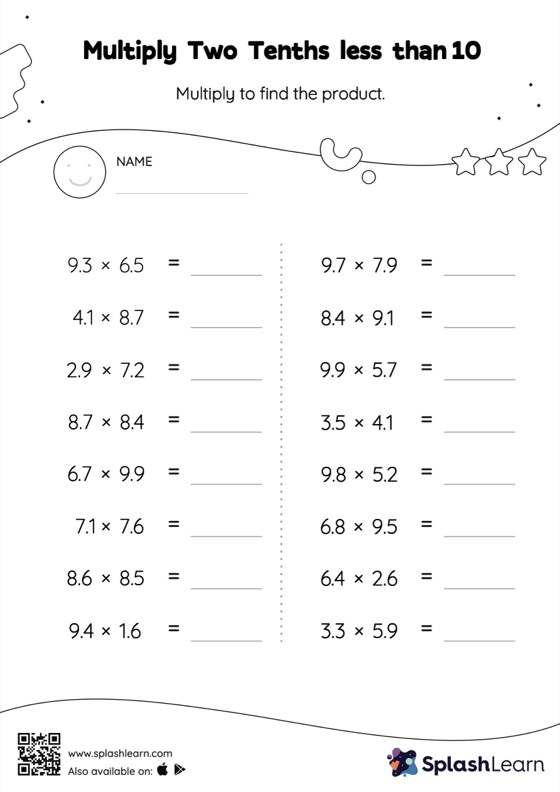# Multiply Two Tenths less than 10: Horizontal Multiplication Worksheet

Home > Multiply Two Tenths less than 10: Horizontal MultiplicationThis worksheet challenges young mathematicians to hone their math skills by solving a set of problems on multiplying two tenths less than 10. When a tenth is multiplied by another tenth, the product will have two decimal digits. Students first multiply the numbers ignoring the decimal points, and then add the decimal point two places from the right in the product. They practice this concept extensively in multiply two tenths less than 10 worksheet.